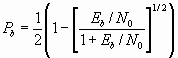### JPL's Wireless Communication Reference Website# BER or SER in a slowly fading channel

Multipath fading channels are notoriously poor. The BER is usually much worse that would occur in stationary channels with the same (average) signal-to-noise ratio. The effect of fading can be quite complicated, as time and frequency dispersion may influence receiver performance. This requires understanding of the delay spread, Doppler spread and scatter function, such that effects like intersymbol interference, synchronization errors due to Doppler can be considered. These mechanisms affect narrowband modulation, spread-spectrum (DS-CDMA, MC-CDMA and FH) and OFDM all in a different manner, as is discussed on the digital modulation page.

Under idealized circumstances however, the average BER can easily be calculated. In a very slowly fading, frequency non-selective channel, the BER can be estimated by simply averaging the BER for a given received signal power (given Eb/N0) over the fading probability distribution. The BER for BPSK in slow Rayleigh fading channel isFigure: (Local-mean) Average Bit Error Rate due to Rician fading versus average signal-to-noise ratio. a: Rayleigh fading b: Rician K factor 4 (6 dB) c: Rician K factor 16 (12 dB) d: No fading (Linear Time Invariant Additive White Gaussian Noise channel); Rician K factor approaching infinity. e: Approximation for large C/I.Figure: Bit Error Rate due to shadowing and Rician fading versus average signal-to-noise ratio. a: shadowing 0 dB; Rician factor 6 dB b: shadowing 6 dB; Rician factor 6 dB c: shadowing 12 dB; Rician factor 6 dB d: shadowing 0 dB; Rician factor 12 dB e: shadowing 6 dB; Rician factor 12 dB f: shadowing 12 dB; Rician factor 12 dB

At large C/I the effect of the Rician factor dominates, while at small C/I the effect of shadowing is more relevant.

As a rule of the thump: BERs often are approximately equal to 0.5 times the outage probability of the link: whenever the received signal is above a certain threshold, the BER is close to zero; when the signal fades below threshold, the BER is about one half.